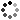# 8th Grade Math Higher Order Thinking Skills Activity - What's the Question

On Sale
\$4.50
\$4.50
Preview

Encourage your 8th grade math students to use higher order thinking skills with this activity. Students will use their critical thinking skills as they develop the question to the answer given.  This product consists of 13 different 8th grade math topics. There is one topic per page. Each topic consists of 5 different “questions.” The questions are actually the answers given to five different problems, and the students have to create the questions. This will help your students think deeper about the mathematics.

The 13 topics include:
• Number Systems: Categorizing rational numbers, integers, whole numbers, irrational numbers, and natural numbers
• Exponents: Simplifying expressions using exponent properties
• Scientific Notation: Writing in scientific notation as well as performing operations on numbers written in scientific notation.
• Equations with Variables on Both Sides
• Systems of Equations
• Functions: Identifying functions from tables, graphs and equations.
• Graphing: Graphing in slope-intercept form.
• Parallel Lines: Identifying angles created by parallel lines cut by a transversal.
• Pythagorean Theorem
• Cones, Cylinders, & Spheres: Finding the volume of these shapes.
• Transformations
• Scatter Plots: Identifying linear, non-linear, positive and negative associations.
An answer sheet is NOT included. Due to the nature of the activity, the answers will widely vary. However, I did include various sample answers. A suggestion for the teacher: do not be so strict on students writing the right “answer” for this activity. Rather this is to challenge students to think beyond basic algorithms. Focus on the thinking of the students and you can learn a great deal on what your students really understand.

These would be great to use for assessments, homework, math centers, group work, or individual work.

Please feel free to contact me with any questions!

Michelle,
Make Sense of Math